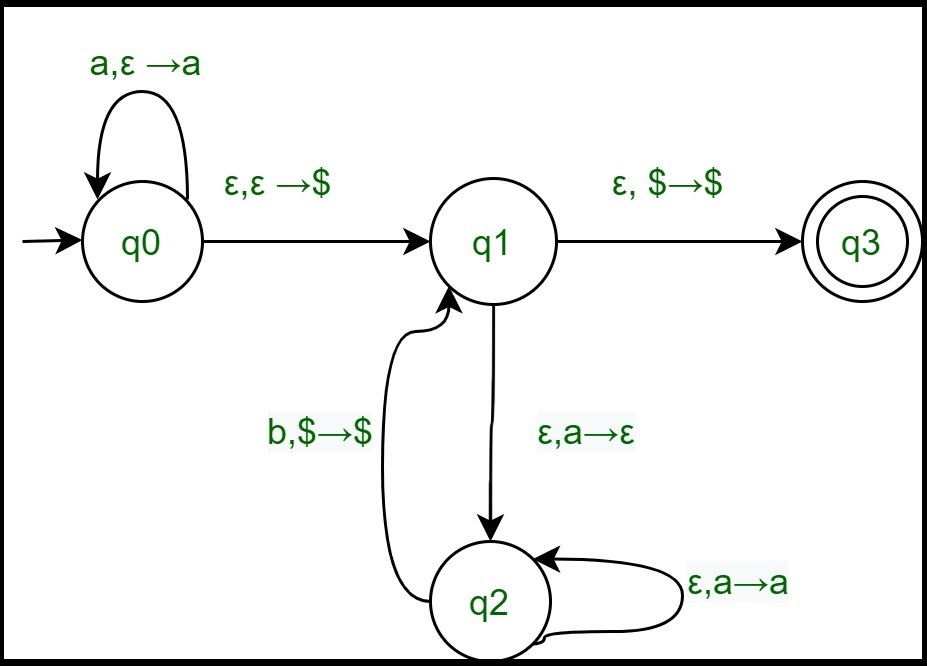# Introduction to Queue Automata

We already know about Finite Automata which can be used to accept regular languages and Pushdown Automata that can be used to recognize Context Free Languages.

Queue Automata(QDA) is a non-deterministic automata that is similar to Pushdown Automata but has a queue instead of a stack which helps Queue automata to recognize languages beyond Context Free Languages.

A QDA is a 6 – tupleWhere

1. Q is the set of finite states.
2.is the set of finite input alphabets.
3.is the set of finite queue alphabets.
4..
5.is the start state.
6. FQ is the set of accept states.

Acceptance of a string

A QDAaccepts inputifcan be written as, where eachand there are statesand stringsexist, such that they satisfy the following conditions:

1.and.
2. Forandandand3.Example:
Define the queue automata for languageSolution:
Q = {q0, q1, q2, q3} and={a, b} and= {a, b, $} And the transition functions are given by:Let us see how this automata works for aabb. Row State Input Transition function Queue(Input from left) State after move 1 q0 aabb δ(q0, a, ε)={(q0, a)} a q0 2 q0 aabb δ(q0, a, ε)={(q0, a)} aa q0 3 q0 ε δ(q0, ε, ε)={(q1,$)} $aa q1 4 q1 ε δ(q1, ε, a)={(q2, ε)}$a q2
5 q2 ε δ(q2, ε, a)={(q2, a)} a$q2 6 q2 aabb δ(q2, b,$)={(q1, $)}$a q1
7 q1 ε δ(q1, ε, a)={(q2, ε)} $q2 8 q2 aabb δ(q2, b,$)={(q1, $)}$ q1
9 q1 ε δ(q1, ε, $)={(q3,$)} \$ q3

Attention reader! Don’t stop learning now. Get hold of all the important CS Theory concepts for SDE interviews with the CS Theory Course at a student-friendly price and become industry ready.

My Personal Notes arrow_drop_up

## Recommended Posts:Check out this Author's contributed articles.

If you like GeeksforGeeks and would like to contribute, you can also write an article using contribute.geeksforgeeks.org or mail your article to contribute@geeksforgeeks.org. See your article appearing on the GeeksforGeeks main page and help other Geeks.

Please Improve this article if you find anything incorrect by clicking on the "Improve Article" button below.

Article Tags :

Be the First to upvote.

Please write to us at contribute@geeksforgeeks.org to report any issue with the above content.